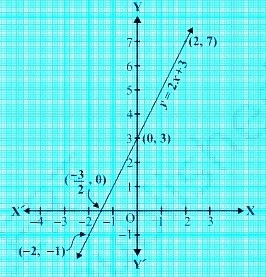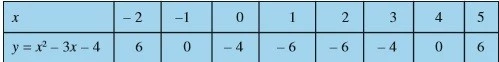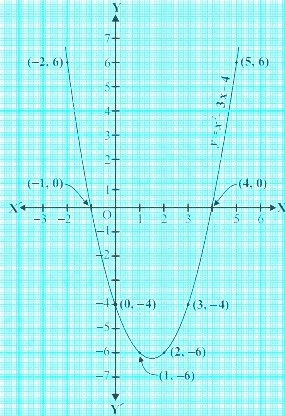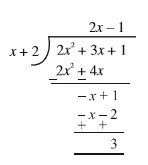# Polynomials NCERT Solutions : Class 10 Maths

## NCERT Solutions for Class 10 Maths : PolynomialsFilter
Filters :
Classes
•
•
•
•
•
•
•
Chapters
•
•
•
•
•
•
•
•
•
•
• 5 More
Exercises
•
•
•
•
•

Doubtnut one of the best online education platform provides free NCERT Solutions of Maths for Class 10 Polynomials which are solved by our maths experts as per the NCERT (CBSE) guidelines. We provide the solutions as video solutions in which concepts are also explained along with answer which will help students while learning , preparing for exams and in doing homework. These Solutions will help to revise complete syllabus and score more marks in examinations.

Get here free, accurate and comprehensive NCERT Solutions for Class 10 Maths Polynomials which have been reviewed by our maths counsellors as per the latest edition following up the CBSE guidelines. We provide video solutions in which solutions to all the questions of NCERT Class 10 Maths textbook are explained in step by step and detailed way.

## The Topics of the Chapter Polynomials are :

RELATIONSHIP B/W THE ZEROES AND COEFF. OF POLYNOMIAL

DIVISION ALGORITHM FOR POLYNOMIALS

GRAPHS OF POLYNOMIALS

It contains these exercises along with solved examples. All the exercises are solved in the video. Select the exercise to view the solutions exercisewise of the Chapter Polynomials:

## NCERT Solutions Class 10 Maths Chapter Polynomials Exercises:

We have covered all the exercises and also Solved examples in the videos. Along with the practise exercise Students should also practise solved examples to clear the concepts of the Polynomials. If incase you have any doubt you can watch the solutions for the given questions.Watch the solutions for the given questions in which it is also explained in the video steps to solve the questions along with answers.

# NCERT Class 10 Maths Solutions Chapter 2 Polynomials

In the previous chapter, we have learned about real numbers. Here in Chapter 2, we will learn about Polynomials which include the geometrical meaning of the zeroes of a polynomial, relationship between zeroes and coefficients of a polynomial and division algorithm for polynomials along with useful examples. This will help the students to get the right idea on how to work on the different problems in the best manner. The team of Doubtnut has made it possible to provide the students with the best and easy approach that helps them to get all their queries cleared. So, getting cleared of all the different topics and subtopics proves to be possible in the right way.

Class 10 is a pivotal mark in the formal education system as it is the first-time students appear for centralized exams conducted by an external authority. The results of CBSE secondary exams help the students in deciding the subjects for the next two years and crafting the future. Students who follow NCERT textbooks find that the basic concepts have been cleared, but when it comes to different tricky questions that are asked in the examination, they find it quite difficult. So, with the hope of scoring good marks by clearing their confusions, they try to search for the best reference books. It is also important to note that if students are not able to get the right concept of Maths, it would truly be impossible for them to achieve success. Therefore, with the best idea on how to attempt different questions with the help of the right procedure, students can always expect to score higher marks in the examinations.

With the aim to make the students get the perfect idea on the different topics and subtopics of NCERT Class 10 Maths Solutions Chapter 2, Doubtnut has introduced online video tutorials. Here, the students are able to find it much easier in clearing their queries in the best easy approach. These study materials have also been prepared in the simplest language so that students do not find it difficult to understand the different topics. It is also possible to get the online study materials downloaded in the pdf format so that they can access it from anywhere without any problem at all.

## Topics and Subtopics included in NCERT Solutions for Class 10 Maths Chapter 2 Polynomials

### Introduction

You have learned about polynomials in Class 9.  It is important to keep in mind that if p(x) is a polynomial in x then the highest power of x in p(x) is known as the degree of the polynomial.  A linear polynomial is a polynomial of degree 1. On the other hand, a polynomial of degree 2 is called a quadratic polynomial. Here, the name, ‘quadratic’ has been derived from the word, ‘quadrate’ which means square.

### Geometrical Meaning of the Zeroes of a Polynomial

It is a known fact that if p(k) =0, then a real number k is a zero of the polynomial p(x). But do you know as to why the zeroes of a polynomial are so important? In order to find out this answer, we need to have a look at the geometrical representations of linear and quadratic polynomials.

Let us consider a linear polynomial ax + b, a ≠ 0. It is known that the graph of y = ax + b is a straight line.you can see that the graph of y = 2x + 3 intersects the x-axis mid-way between x = –1 and x = –2, that is, at the point -3, 0

2

You are also familiar that the zero of 2x + 3 is -3

2

Thus, the zero of the polynomial 2x + 3 is the x-coordinate of the point where the graph of y = 2x + 3 intersects the x-axis. In general, for a linear polynomial ax + b, a ≠ 0, the graph of y = ax + b is a straight line which intersects the x-axis at exactly one point, namely –b, 0

a

Therefore, the linear polynomial ax + b, a ≠ 0, has exactly one zero, namely, the x-coordinate of the point where the graph of y = ax + b intersects the x-axis.

Now, let us look for the geometrical meaning of a zero of a quadratic polynomial. Consider the quadratic polynomial x2 – 3x – 4. Let us see what the graph* of y = x2 – 3x – 4 looks like. Let us list a few values of y = x2 – 3x – 4 corresponding to a few values for x.If we locate the points listed above on a graph paper and draw the graph, it will actually look like the one as below:For any quadratic polynomial ax2 + bx + c, a ≠ 0, the graph of the corresponding equation y = ax2 + bx + c has one of the two shapes either open upwards or open downwards depending on whether a > 0 or a < 0. (These curves are called parabolas.)

You can see from the table that –1 and 4 are zeroes of the quadratic polynomial. Also, note from the table that –1 and 4 are the x-coordinates of the points where the graph of y = x2 – 3x – 4 intersects the x-axis. Thus, the zeroes of the quadratic polynomial x2 – 3x – 4 are x-coordinates of the points where the graph of y = x2 – 3x – 4 intersects the x-axis.

This fact is true for any quadratic polynomial, i.e., the zeroes of a quadratic polynomial ax2 + bx + c, a ≠ 0, are precisely the x-coordinates of the points where the parabola representing y = ax2 + bx + c intersects the x-axis.

### Relationship between Zeroes and Coefficients of a Polynomial

It has been learned that zero of a linear polynomials ax + b is - b

a

Let’s take an example:

Find the zeroes of the quadratic polynomial x2 + 7x + 10, and verify the relationship between the zeroes and the coefficients.

Solution: We have

x2 + 7x + 10 = (x + 2)(x + 5)

So, the value of x2 + 7x + 10 is zero when x + 2 = 0 or x + 5 = 0, i.e., when x = – 2 or x = –5. Therefore, the zeroes of x2 + 7x + 10 are – 2 and – 5. Now,

Sum of zeroes = (-2) + (-5) = -(7) = - (Coefficient of x)

1         Coefficient of x2

Product of zeroes = (-2) x (-5) =10 = 10 =    Constant term

1        Coefficient of x2

### Division Algorithm for Polynomials

It has been learned that a cubic polynomial has at most three zeroes. Suppose you are given only one zero, how can you find the other two? For this, let us consider the cubic polynomial x3 – 3x2 – x + 3. If we tell you that one of its zeroes is 1, then you know that x – 1 is a factor of x3 – 3x2 – x + 3. So, you can divide x3 – 3x2 – x + 3 by x – 1, as you have learned in Class IX, to get the quotient x2 – 2x – 3.

Next, you could get the factors of x2 – 2x – 3, by splitting the middle term, as (x + 1)(x – 3). This would give you x3 – 3x2 – x + 3 = (x – 1)(x2 – 2x – 3) = (x – 1)(x + 1)(x – 3)

So all the three zeroes of the cubic polynomial are now known to you as 1, – 1, 3.

Let’s take an example:

Divide 2x2 + 3x + 1 by x + 2.

Solution: Note that we stop the division process when either the remainder is zero or its degree is less than the degree of the divisor. So, here the quotient is 2x – 1 and the remainder is 3. Also, (2x – 1)(x + 2) + 3 = 2x2 + 3x – 2 + 3 = 2x2 + 3x + 1 i.e., 2x2 + 3x + 1 = (x + 2)(2x – 1) + 3. Therefore, Dividend = Divisor × Quotient + Remainder## Why is Doubtnut useful for Class 10 students?

Doubtnut proves to be of much use for Class 10 students as it has revolutionized the method of imparting the best education to the students. So, let’s find out its usefulness in order to get the right idea about it.

• Lots of exercises and examples included: The team of expert tutors of Doubtnut has included lots of exercises and examples for students for which it proves to be quite useful to them. Thus, also helps them to get the right idea on how to attempt even the toughest questions that are set in the examination papers. It is also possible to get the most important Class 10 Maths NCERT Solutions that truly proves to be of much use. So, students get the right grip of the knowledge on how to come up with the perfect solution without any problem at all.
• Free access: Another advantage that the students can enjoy is the free access provided by Doubtnut. It does not lead to spending any money on accessing different and useful online study materials. So, by making the best choice to approach them would definitely help a lot to save both money and time.  It can help in boosting confidence that proves to be quite important for the students to achieve success.
• Provides step by step methodology: Doubtnut’s video tutorials provide step by step instructions to the students which helps a lot to get cleared of all the important topics. This makes it much easier for them to get the perfect idea as to how to attempt any questions without any worry. This also makes the students to develop a liking or interest for the subject as well. So, achieving success in exams is possible by opting for the best and useful online study materials provided by Doubtnut.
• Effective for competitive exam preparation: As in recent times the education system has become a rather competitive place for the students. They no longer just have their academic exams to worry about or prepare for, but also appear in a number of other competition exams. The student also prepares for talent hunt exams organized on a national level, such as NTSE and Olympiads. The book RD Sharma helps the students with their preparations for these competitive exams, provided that they are aware of the syllabus and curriculum for the exams.  Moreover, it helps in building solid foundation for future engineering entrance exams like IIT JEE Mains and Advanced.

Share this on :-